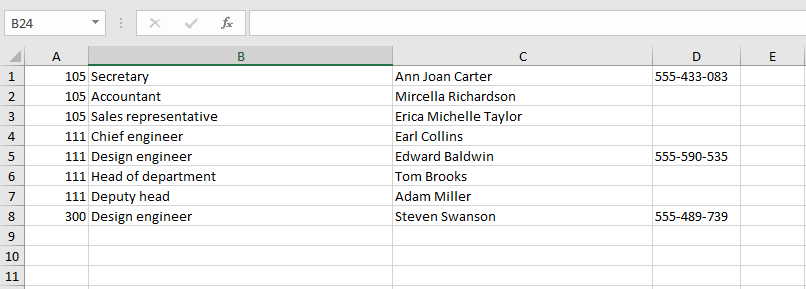You can load users from a file with the following structure: columns with data are on the first sheet (name of the sheet is irrelevant); the first column contains the employee's office number; the second - job position (this information will be skipped); the third column contains the full name of the employee; the fourth column contains the work phone number. One row in the file stands for one uploaded user. The information encoding in the file by default corresponds to the code page 1251 and does not require transformation.

Take into account, that the number of uploaded users should not be greater than the number of user licenses.

## File structure exampleFor the script to work correctly, add the namespaces:

``````using Aspose.Cells;
using EleWise.ELMA.Security.Managers;``````
Script:
``````int current_row   = 0;   //  current row of the Excel file sheet
int current_sheet = 0;   //  current sheet. Starts with 0
int current_column= 0;   //  current column

if (context.SourceFile == null)
{
throw new Exception(SR.T("Source file is not selected!"));
return;
}

//  get the link to the file from a context variable
string filepath_excel = context.SourceFile.ContentFilePath;

// load excel file data to the object
var Excelbook  = new Workbook(filepath_excel);
// set to the sheet 1, which will be the data source
var Excelsheet = Excelbook.Worksheets[current_sheet];

//  check if the Full Name field is empty; if it is, then finish the cycle
while (Excelsheet.Cells[current_row, current_column+2].StringValue != "")
{
//  parse the field value into an array:  Last Name (stfio),  Name (stfio),  Middle Name (stfio)
string[]  stfio    =  Excelsheet.Cells[current_row, current_column+2].StringValue.Split(’ ’);

// Create an instance of the User object
var UserNew        = UserManager.Instance.Create();
UserNew.FirstName  = stfio;         //  name
UserNew.MiddleName = stfio;         //  middle name
UserNew.LastName   = stfio;         //  last name

//  account or login for signing in to the system (miller)
UserNew.UserName   = stfio + stfio.Substring(0,1) +stfio.Substring(0,1);

UserNew.FullName   = Excelsheet.Cells[current_row, current_sheet+2].StringValue; // full name
UserNew.RoomNumber = Excelsheet.Cells[current_row, current_sheet].StringValue;   //  room number
UserNew.WorkPhone  = Excelsheet.Cells[current_row, current_sheet+3].StringValue; //  phone number

// save the object instance
UserNew.Save();

//  row counter increment
current_row = current_row + 1;

}``````